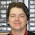## Friday, November 6, 2015

### HP Prime Geometry App Tutorial Part 2: Triangles

HP Prime Geometry App Tutorial Part 2:  Triangles

Like Part 1, we’ll use a Plot window of XRange = [ -16, 16 ], YRange = [ -11, 10.9 ], ticks are at 1.  For this lesson, we are going to focus on triangles.  Start with a clear Plot Screen ([Shift], [ Esc ] (Clear)).

Drawing a Triangle

1.  On the plot screen, press the soft key (Cmds), then select 4 for Polygon and 1 for Triangle.
2.  Select the first vertex (corner point) and press [ Enter ].  For this lesson, put the triangle wherever you want.
3.  Place the second and third vertex.  Press [ Enter ] after each point.  The vertices are labeled A, B, and C.

Finding an Angle

The steps will demonstrate how to find the angle.  Be aware that the direction you enter the vertexes will determine the sign of the angle.

1.  Press (Cmds), 9 for Measure, 6 for Angle.
2.  Select one vertex, press [Enter].  Follow the triangle to select the second vertex (where the angle will be measured), press [ Enter ].  Follow that with a third vertex and press [Enter].  The angle displayed on top of the screen.

Resizing the Triangle

Click on one of the points of the points.  Then drag the point with the arrow pad.  When satisfied, press [ Enter ].

For the last part, clear the screen.

Reflect a Triangle – reflect about a point

1.  Clear the screen.  ([ Shift ] [ Esc ] (Clear))
2.  Draw a triangle with the vertices (-6, -4), (-6, 4), and (-12, 0).
3.  Press (Cmds), 7 for Transform, 2 for Reflection.
4.  You will be promoted for a reflection point.  For this exercise, set the point at (0,0).
5.  Select the triangle (scroll until the triangle turns red) and press [ Enter ].

In Part 3, we’re going to work with lines and line segments.  Until next time, have a great day!

Eddie

This blog is property of Edward Shore.  2015.

1.2.